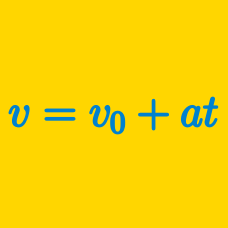Classical Mechanics

# Position Vector for Constant Acceleration

If an object is thrown straight upward with an initial speed of $$8\text{ m/s}$$ and takes $$7$$ seconds to hit the ground, from what height was the object thrown?

Assume that gravitational acceleration is $$g=10\text{ m/s}^2.$$

A race car is on a straight track. The race car accelerates from rest, covering $$100~\mbox{m}$$ in $$5~\mbox{seconds}$$. What is the acceleration of the race car in $$\mbox{m/s}^2$$?

Details and assumptions

• You may neglect air resistance.

A rock is dropped vertically off the edge of a cliff and hits the ground with an impact velocity of $$90\text{ m/s}.$$ How high is the cliff?

The gravitational acceleration is $$g=10\text{ m/s}^2.$$

An object initially at rest starts moving in a straight line, accelerating uniformly at $$3\text{ m/s}^2$$ for $$2$$ seconds. Then it moves with a constant acceleration of $$-2\text{ m/s}^2$$ for $$8$$ seconds, and then it accelerates uniformly at $$1\text{ m/s}^2$$ for $$8$$ seconds. Find the displacement of the object during the total $$18$$ seconds.

Cliff diving is an extreme sport. If a cliff diver dives from a platform 50 meters above the water, how long does the dive take in seconds?

Details and assumptions

• The acceleration of gravity is $$-9.8~\mbox{m/s}^2$$.
• The diver can be treated as a point mass and has no vertical velocity at the start of the dive.
• Ignore air resistance.
×

Problem Loading...

Note Loading...

Set Loading...